# Mean State

Period Mean (original grids) [Watt m-2]
Model Period Mean (intersection) [Watt m-2]
Model Period Mean (complement) [Watt m-2]
Benchmark Period Mean (intersection) [Watt m-2]
Benchmark Period Mean (complement) [Watt m-2]
Bias [Watt m-2]
RMSE [Watt m-2]
Phase Shift [months]
Bias Score 
RMSE Score 
Seasonal Cycle Score 
Spatial Distribution Score 
Interannual Variability Score 
Overall Score 
Benchmark [-] 122.
CLM5PHSOFF [-] 116. 116. 0.00 120. 164. -4.34 18.2 1.11 0.67 0.43 0.85 0.91 0.69 0.67
CLM5PHSON [-] 116. 116. 0.00 120. 164. -3.73 17.7 0.987 0.68 0.44 0.87 0.89 0.67 0.67
Period Mean (original grids) [Watt m-2]
Model Period Mean (intersection) [Watt m-2]
Model Period Mean (complement) [Watt m-2]
Benchmark Period Mean (intersection) [Watt m-2]
Benchmark Period Mean (complement) [Watt m-2]
Bias [Watt m-2]
RMSE [Watt m-2]
Phase Shift [months]
Bias Score 
RMSE Score 
Seasonal Cycle Score 
Spatial Distribution Score 
Interannual Variability Score 
Overall Score 
Benchmark [-] 102.
CLM5PHSOFF [-] 96.4 95.9 0.00 101. 165. -4.73 20.0 1.24 0.66 0.51 0.81 0.86 0.69 0.68
CLM5PHSON [-] 97.7 97.2 0.00 101. 165. -3.30 19.8 1.28 0.67 0.51 0.81 0.89 0.65 0.67
Period Mean (original grids) [Watt m-2]
Model Period Mean (intersection) [Watt m-2]
Model Period Mean (complement) [Watt m-2]
Benchmark Period Mean (intersection) [Watt m-2]
Benchmark Period Mean (complement) [Watt m-2]
Bias [Watt m-2]
RMSE [Watt m-2]
Phase Shift [months]
Bias Score 
RMSE Score 
Seasonal Cycle Score 
Spatial Distribution Score 
Interannual Variability Score 
Overall Score 
Benchmark [-] 112.
CLM5PHSOFF [-] 104. 103. 0.00 110. 159. -6.56 21.3 0.873 0.68 0.57 0.88 0.85 0.65 0.70
CLM5PHSON [-] 105. 105. 0.00 110. 159. -3.73 20.8 1.22 0.71 0.57 0.81 0.87 0.61 0.69
Period Mean (original grids) [Watt m-2]
Model Period Mean (intersection) [Watt m-2]
Model Period Mean (complement) [Watt m-2]
Benchmark Period Mean (intersection) [Watt m-2]
Benchmark Period Mean (complement) [Watt m-2]
Bias [Watt m-2]
RMSE [Watt m-2]
Phase Shift [months]
Bias Score 
RMSE Score 
Seasonal Cycle Score 
Spatial Distribution Score 
Interannual Variability Score 
Overall Score 
Benchmark [-] 43.3
CLM5PHSOFF [-] -1.69 29.0 0.00 43.2 44.3 -14.5 25.8 0.239 0.77 0.68 0.98 0.79 0.76 0.78
CLM5PHSON [-] 33.3 33.3 0.00 43.2 44.3 -11.6 23.4 0.172 0.81 0.70 0.99 0.78 0.76 0.79
Period Mean (original grids) [Watt m-2]
Model Period Mean (intersection) [Watt m-2]
Model Period Mean (complement) [Watt m-2]
Benchmark Period Mean (intersection) [Watt m-2]
Benchmark Period Mean (complement) [Watt m-2]
Bias [Watt m-2]
RMSE [Watt m-2]
Phase Shift [months]
Bias Score 
RMSE Score 
Seasonal Cycle Score 
Spatial Distribution Score 
Interannual Variability Score 
Overall Score 
Benchmark [-] 144.
CLM5PHSOFF [-] 133. 132. 0.00 140. 160. -7.53 20.6 1.07 0.60 0.42 0.84 0.89 0.61 0.63
CLM5PHSON [-] 128. 127. 0.00 140. 160. -7.88 20.6 0.968 0.60 0.43 0.86 0.90 0.60 0.64
Period Mean (original grids) [Watt m-2]
Model Period Mean (intersection) [Watt m-2]
Model Period Mean (complement) [Watt m-2]
Benchmark Period Mean (intersection) [Watt m-2]
Benchmark Period Mean (complement) [Watt m-2]
Bias [Watt m-2]
RMSE [Watt m-2]
Phase Shift [months]
Bias Score 
RMSE Score 
Seasonal Cycle Score 
Spatial Distribution Score 
Interannual Variability Score 
Overall Score 
Benchmark [-] 62.5
CLM5PHSOFF [-] 42.8 42.7 0.00 62.3 75.7 -19.6 28.1 0.105 0.71 0.70 0.99 0.96 0.66 0.79
CLM5PHSON [-] 48.1 48.1 0.00 62.3 75.7 -13.9 23.3 0.107 0.79 0.72 0.99 0.94 0.67 0.80
Period Mean (original grids) [Watt m-2]
Model Period Mean (intersection) [Watt m-2]
Model Period Mean (complement) [Watt m-2]
Benchmark Period Mean (intersection) [Watt m-2]
Benchmark Period Mean (complement) [Watt m-2]
Bias [Watt m-2]
RMSE [Watt m-2]
Phase Shift [months]
Bias Score 
RMSE Score 
Seasonal Cycle Score 
Spatial Distribution Score 
Interannual Variability Score 
Overall Score 
Benchmark [-] 72.7
CLM5PHSOFF [-] 62.2 62.0 0.00 72.1 94.9 -10.1 22.2 0.376 0.77 0.65 0.97 0.99 0.63 0.78
CLM5PHSON [-] 62.7 62.6 0.00 72.1 94.9 -8.19 21.7 0.393 0.79 0.65 0.97 0.99 0.63 0.78
Period Mean (original grids) [Watt m-2]
Model Period Mean (intersection) [Watt m-2]
Model Period Mean (complement) [Watt m-2]
Benchmark Period Mean (intersection) [Watt m-2]
Benchmark Period Mean (complement) [Watt m-2]
Bias [Watt m-2]
RMSE [Watt m-2]
Phase Shift [months]
Bias Score 
RMSE Score 
Seasonal Cycle Score 
Spatial Distribution Score 
Interannual Variability Score 
Overall Score 
Benchmark [-] 109.
CLM5PHSOFF [-] 96.5 95.7 0.00 105. 157. -9.55 24.3 0.467 0.68 0.54 0.96 0.96 0.57 0.71
CLM5PHSON [-] 94.4 94.1 0.00 105. 157. -6.92 23.7 0.544 0.72 0.53 0.95 0.96 0.56 0.71
Period Mean (original grids) [Watt m-2]
Model Period Mean (intersection) [Watt m-2]
Model Period Mean (complement) [Watt m-2]
Benchmark Period Mean (intersection) [Watt m-2]
Benchmark Period Mean (complement) [Watt m-2]
Bias [Watt m-2]
RMSE [Watt m-2]
Phase Shift [months]
Bias Score 
RMSE Score 
Seasonal Cycle Score 
Spatial Distribution Score 
Interannual Variability Score 
Overall Score 
Benchmark [-] 41.8
CLM5PHSOFF [-] 22.6 23.0 0.00 41.9 41.5 -18.8 24.3 0.236 0.71 0.69 0.98 0.95 0.75 0.80
CLM5PHSON [-] 25.1 25.4 0.00 41.9 41.5 -17.5 23.3 0.253 0.72 0.70 0.98 0.94 0.75 0.80
Period Mean (original grids) [Watt m-2]
Model Period Mean (intersection) [Watt m-2]
Model Period Mean (complement) [Watt m-2]
Benchmark Period Mean (intersection) [Watt m-2]
Benchmark Period Mean (complement) [Watt m-2]
Bias [Watt m-2]
RMSE [Watt m-2]
Phase Shift [months]
Bias Score 
RMSE Score 
Seasonal Cycle Score 
Spatial Distribution Score 
Interannual Variability Score 
Overall Score 
Benchmark [-] 96.5
CLM5PHSOFF [-] 92.9 92.8 0.00 93.2 138. -0.576 20.1 0.376 0.78 0.67 0.97 0.85 0.66 0.77
CLM5PHSON [-] 92.5 92.4 0.00 93.2 138. 1.15 20.2 0.330 0.78 0.67 0.97 0.87 0.64 0.76
Period Mean (original grids) [Watt m-2]
Model Period Mean (intersection) [Watt m-2]
Model Period Mean (complement) [Watt m-2]
Benchmark Period Mean (intersection) [Watt m-2]
Benchmark Period Mean (complement) [Watt m-2]
Bias [Watt m-2]
RMSE [Watt m-2]
Phase Shift [months]
Bias Score 
RMSE Score 
Seasonal Cycle Score 
Spatial Distribution Score 
Interannual Variability Score 
Overall Score 
Benchmark [-] 64.4
CLM5PHSOFF [-] 54.5 54.4 0.00 61.9 90.5 -7.54 19.0 0.276 0.85 0.75 0.98 0.99 0.63 0.82
CLM5PHSON [-] 54.4 54.4 0.00 61.9 90.5 -5.49 19.0 0.289 0.86 0.74 0.98 0.98 0.64 0.82
Period Mean (original grids) [Watt m-2]
Model Period Mean (intersection) [Watt m-2]
Model Period Mean (complement) [Watt m-2]
Benchmark Period Mean (intersection) [Watt m-2]
Benchmark Period Mean (complement) [Watt m-2]
Bias [Watt m-2]
RMSE [Watt m-2]
Phase Shift [months]
Bias Score 
RMSE Score 
Seasonal Cycle Score 
Spatial Distribution Score 
Interannual Variability Score 
Overall Score 
Benchmark [-] 115.
CLM5PHSOFF [-] 72.8 65.5 0.00 77.4 133. -12.0 24.6 0.547 0.66 0.60 0.94 0.93 0.63 0.72
CLM5PHSON [-] 65.7 65.7 0.00 77.4 133. -10.1 23.7 0.563 0.68 0.60 0.93 0.92 0.62 0.73
Period Mean (original grids) [Watt m-2]
Model Period Mean (intersection) [Watt m-2]
Model Period Mean (complement) [Watt m-2]
Benchmark Period Mean (intersection) [Watt m-2]
Benchmark Period Mean (complement) [Watt m-2]
Bias [Watt m-2]
RMSE [Watt m-2]
Phase Shift [months]
Bias Score 
RMSE Score 
Seasonal Cycle Score 
Spatial Distribution Score 
Interannual Variability Score 
Overall Score 
Benchmark [-] 54.9
CLM5PHSOFF [-] 55.7 55.6 0.00 51.3 143. 4.35 25.9 0.893 0.67 0.52 0.89 0.84 0.55 0.66
CLM5PHSON [-] 54.2 54.2 0.00 51.3 143. 5.15 26.1 0.880 0.66 0.52 0.89 0.85 0.55 0.67
Period Mean (original grids) [Watt m-2]
Model Period Mean (intersection) [Watt m-2]
Model Period Mean (complement) [Watt m-2]
Benchmark Period Mean (intersection) [Watt m-2]
Benchmark Period Mean (complement) [Watt m-2]
Bias [Watt m-2]
RMSE [Watt m-2]
Phase Shift [months]
Bias Score 
RMSE Score 
Seasonal Cycle Score 
Spatial Distribution Score 
Interannual Variability Score 
Overall Score 
Benchmark [-] 44.9
CLM5PHSOFF [-] 24.9 24.8 0.00 44.8 45.6 -20.0 25.9 0.133 0.70 0.71 0.99 0.90 0.78 0.80
CLM5PHSON [-] 28.4 28.3 0.00 44.8 45.6 -18.6 24.6 0.122 0.72 0.72 0.99 0.87 0.78 0.80
Period Mean (original grids) [Watt m-2]
Model Period Mean (intersection) [Watt m-2]
Model Period Mean (complement) [Watt m-2]
Benchmark Period Mean (intersection) [Watt m-2]
Benchmark Period Mean (complement) [Watt m-2]
Bias [Watt m-2]
RMSE [Watt m-2]
Phase Shift [months]
Bias Score 
RMSE Score 
Seasonal Cycle Score 
Spatial Distribution Score 
Interannual Variability Score 
Overall Score 
Benchmark [-] 134.
CLM5PHSOFF [-] 110. 111. 0.00 127. 169. -16.2 25.3 0.736 0.61 0.60 0.91 0.95 0.64 0.72
CLM5PHSON [-] 107. 108. 0.00 127. 169. -14.0 24.1 0.799 0.64 0.60 0.90 0.95 0.62 0.72
Period Mean (original grids) [Watt m-2]
Model Period Mean (intersection) [Watt m-2]
Model Period Mean (complement) [Watt m-2]
Benchmark Period Mean (intersection) [Watt m-2]
Benchmark Period Mean (complement) [Watt m-2]
Bias [Watt m-2]
RMSE [Watt m-2]
Phase Shift [months]
Bias Score 
RMSE Score 
Seasonal Cycle Score 
Spatial Distribution Score 
Interannual Variability Score 
Overall Score 
Benchmark [-] 99.6
CLM5PHSOFF [-] 80.3 80.2 0.00 97.8 136. -17.6 24.1 0.345 0.70 0.72 0.97 0.99 0.63 0.79
CLM5PHSON [-] 82.0 82.1 0.00 97.8 136. -14.0 21.5 0.352 0.75 0.72 0.97 1.0 0.62 0.80
Period Mean (original grids) [Watt m-2]
Model Period Mean (intersection) [Watt m-2]
Model Period Mean (complement) [Watt m-2]
Benchmark Period Mean (intersection) [Watt m-2]
Benchmark Period Mean (complement) [Watt m-2]
Bias [Watt m-2]
RMSE [Watt m-2]
Phase Shift [months]
Bias Score 
RMSE Score 
Seasonal Cycle Score 
Spatial Distribution Score 
Interannual Variability Score 
Overall Score 
Benchmark [-] 72.2
CLM5PHSOFF [-] 53.5 53.7 0.00 72.0 77.2 -18.4 25.3 0.109 0.73 0.74 0.99 0.99 0.67 0.81
CLM5PHSON [-] 58.6 58.7 0.00 72.0 77.2 -13.2 20.6 0.102 0.79 0.76 0.99 1.0 0.67 0.83
Period Mean (original grids) [Watt m-2]
Model Period Mean (intersection) [Watt m-2]
Model Period Mean (complement) [Watt m-2]
Benchmark Period Mean (intersection) [Watt m-2]
Benchmark Period Mean (complement) [Watt m-2]
Bias [Watt m-2]
RMSE [Watt m-2]
Phase Shift [months]
Bias Score 
RMSE Score 
Seasonal Cycle Score 
Spatial Distribution Score 
Interannual Variability Score 
Overall Score 
Benchmark [-] 81.6
CLM5PHSOFF [-] 69.1 69.4 0.00 81.1 116. -11.6 22.0 0.312 0.76 0.71 0.98 0.99 0.66 0.80
CLM5PHSON [-] 72.0 72.2 0.00 81.1 116. -8.22 20.4 0.314 0.79 0.71 0.98 0.99 0.66 0.81
Period Mean (original grids) [Watt m-2]
Model Period Mean (intersection) [Watt m-2]
Model Period Mean (complement) [Watt m-2]
Benchmark Period Mean (intersection) [Watt m-2]
Benchmark Period Mean (complement) [Watt m-2]
Bias [Watt m-2]
RMSE [Watt m-2]
Phase Shift [months]
Bias Score 
RMSE Score 
Seasonal Cycle Score 
Spatial Distribution Score 
Interannual Variability Score 
Overall Score 
Benchmark [-] 132.
CLM5PHSOFF [-] 122. 122. 0.00 131. 178. -8.95 21.0 0.945 0.60 0.39 0.87 0.96 0.62 0.64
CLM5PHSON [-] 122. 122. 0.00 131. 178. -7.96 20.3 0.991 0.62 0.39 0.86 0.95 0.60 0.64
Period Mean (original grids) [Watt m-2]
Model Period Mean (intersection) [Watt m-2]
Model Period Mean (complement) [Watt m-2]
Benchmark Period Mean (intersection) [Watt m-2]
Benchmark Period Mean (complement) [Watt m-2]
Bias [Watt m-2]
RMSE [Watt m-2]
Phase Shift [months]
Bias Score 
RMSE Score 
Seasonal Cycle Score 
Spatial Distribution Score 
Interannual Variability Score 
Overall Score 
Benchmark [-] 117.
CLM5PHSOFF [-] 106. 106. 0.00 117. 145. -10.9 21.6 0.959 0.68 0.55 0.87 0.98 0.65 0.71
CLM5PHSON [-] 108. 108. 0.00 117. 145. -8.04 20.3 0.874 0.73 0.56 0.88 0.97 0.62 0.72
Period Mean (original grids) [Watt m-2]
Model Period Mean (intersection) [Watt m-2]
Model Period Mean (complement) [Watt m-2]
Benchmark Period Mean (intersection) [Watt m-2]
Benchmark Period Mean (complement) [Watt m-2]
Bias [Watt m-2]
RMSE [Watt m-2]
Phase Shift [months]
Bias Score 
RMSE Score 
Seasonal Cycle Score 
Spatial Distribution Score 
Interannual Variability Score 
Overall Score 
Benchmark [-] 95.7
CLM5PHSOFF [-] 88.6 87.8 0.00 92.5 124. -4.73 25.3 0.388 0.73 0.68 0.96 0.99 0.56 0.77
CLM5PHSON [-] 90.8 90.1 0.00 92.5 124. -3.40 24.9 0.391 0.74 0.68 0.96 0.99 0.57 0.77

# Temporally integrated period mean

BENCHMARK MEAN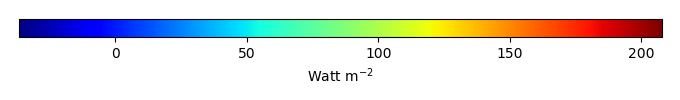MODEL MEANBIAS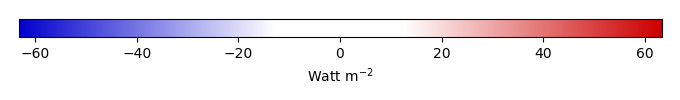BIAS SCORERMSE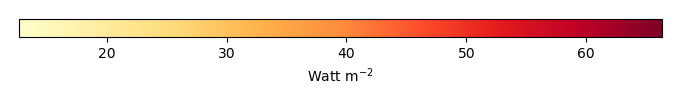RMSE SCOREBENCHMARK INTERANNUAL VARIABILITY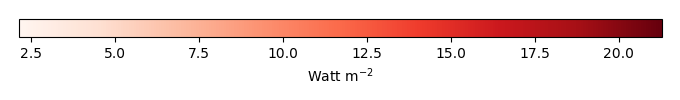MODEL INTERANNUAL VARIABILITYINTERANNUAL VARIABILITY SCOREBENCHMARK MAX MONTHMODEL MAX MONTHDIFFERENCE IN MAX MONTH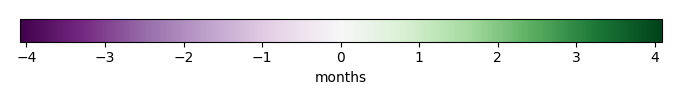SEASONAL CYCLE SCORESPATIAL TAYLOR DIAGRAMMODEL COLORS# Spatially integrated regional mean

MODEL COLORSREGIONAL MEANANNUAL CYCLEMONTHLY ANOMALYANNUAL CYCLE# All Models

BenchmarkCLM5PHSOFFCLM5PHSON# Data Information

creation_date: Tue Jul 1 08:16:58 PDT 2014

source_file: This product is generated from monthly 1 degree GEWEX SRB Radiation observations